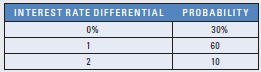# Probability Distribution of Forecasts Assume that the following regression model was applied to historical quarterly data: e t = a 0 + a 1 I N T t + a 2 I N F t − 1 + μ t where: e t = percentage change in the exchange rate of the Japanese yen in period t INT t = average real interest rate differential (U.S. interest rate minus Japanese interest rate) over period t INF t − 1 = inflation differential (U.S. inflation rate minus Japanese inflation rate) in the previous period a 0 , a 1 , a 2 = regression coefficients μ t = error term Assume that the regression coefficients were estimated as follows: a 0 = 0.0 a 1 = 0.9 a 2 = 0.8 Also assume that the inflation differential in the most recent period was 3 percent. The real interest rate differential in the upcoming period is forecasted as follows: If Stillwater, Inc., uses this information to forecast the Japanese yen’s exchange rate, what will be the probability distribution of the yen’s percentage change over the upcoming period?FindFind

### International Financial Management

14th Edition
Madura
Publisher: Cengage
ISBN: 9780357130698FindFind

### International Financial Management

14th Edition
Madura
Publisher: Cengage
ISBN: 9780357130698

#### Solutions

Chapter 9, Problem 19QA
Textbook Problem

## Probability Distribution of Forecasts Assume that the following regression model was applied to historical quarterly data: e t = a 0 + a 1 I N T t + a 2 I N F t − 1 + μ t where: e t = percentage change in the exchange rate of the Japanese yen in period t INT t = average real interest rate differential (U.S. interest rate minus Japanese interest rate) over period t INF t − 1 = inflation differential (U.S. inflation rate minus Japanese inflation rate) in the previous period a 0 , a 1 , a 2 = regression coefficients μ t = error termAssume that the regression coefficients were estimated as follows: a 0 = 0.0 a 1 = 0.9 a 2 = 0.8 Also assume that the inflation differential in the most recent period was 3 percent. The real interest rate differential in the upcoming period is forecasted as follows:If Stillwater, Inc., uses this information to forecast the Japanese yen’s exchange rate, what will be the probability distribution of the yen’s percentage change over the upcoming period?

This textbook solution is under construction.

## Expert Solution

### Want to see the full answer?

Check out a sample textbook solution.

### Want to see this answer and more?

Experts are waiting 24/7 to provide step-by-step solutions in as fast as 30 minutes!*

*Response times vary by subject and question complexity. Median response time is 34 minutes and may be longer for new subjects.© 2021 bartleby. All Rights Reserved.